# De Boor's AlgorithmDe Boor's algorithm is a generalization of de Casteljau's algorithm. It provides a fast and numerically stable way for finding a point on a B-spline curve given a u in the domain.

Recall from a property of multiple knots that increasing the multiplicity of an internal knot decreases the number of non-zero basis functions at this knot. In fact, if the multiplicity of this knot is k, there are at most p - k + 1 non-zero basis functions at this knot. Consequently, at a knot of multiplicity p, there will be only one non-zero basis function whose value at this knot is one because of the property of partition of unity. Let this knot be ui. If u is ui, since Ni,p(u) is non-zero on [ui,ui+1), the point on the curve p(u) is affected by exactly one control point pi. More precisely, we actually have p(u) = Ni,p(u) pi = pi!

So, what is the point of this interesting or somewhat strange property? Simple: if a knot u is inserted p times to a B-spline/NURBS curve, the last generated new control point is the point on the curve that corresponds to u. Why is this so? After inserting u p times, the triangular computation scheme yields one point. Because the given B-spline/NURBS curve must passe this new point, it is the point on the curve corresponding to u. Note that this argument holds even if u is inserted as an existing knot.

Therefore, this observation provides us with a technique for finding p(u) on the curve. We just simply insert u p times and the last point is p(u)!

Let us take a look at an example before move on. The following is a degree 4 NURBS curve with 7 control point. To compute p(0.9), u = 0.9 is inserted 4 (the degree) times. The second and third figures show the results after the first and second insertion. Thus, two new control points are added near the lower right corner. Please note that the control polyline is closer to the curve than the original. The result of the third insertion is shown in the fourth. We have one more control point; but the point on curve is not yet numbered since it is not yet a control point. The fourth insertion makes this happen. Therefore, after four insertion, p(0.9) is a control point.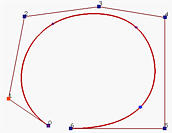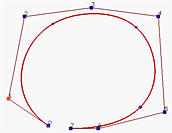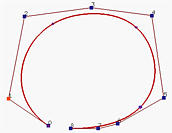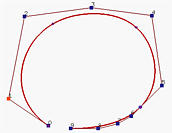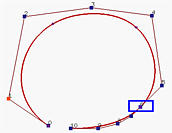The general knot insertion algorithm discussed on the previous page can be easily modified to fulfill our purpose. First note that we only need to insert u enough number of times so that u becomes a knot of multiplicity p. If u is already a knot of multiplicity s, then inserting it p - s times would be sufficient.

Input: a value u
Output: the point on the curve, p(u)

If u lies in [uk,uk+1) and u != uk, let h = p (i.e., inserting u p times) and s = 0;
If u = uk and uk is a knot of multiplicity s, let h = p - s (i.e., inserting u p - s time);
Copy the affected control points pk-s, pk-s-1, pk-s-2, ..., pk-p+1 and pk-p to a new array and rename them as pk-s,0, pk-s-1,0, pk-s-2,0, ..., pk-p+1,0;

for r := 1 to h do
for i := k-p+r to k-s do
begin
Let ai,r = (u - ui) / ( ui+p-r+1 - ui )
Let pi,r = (1 - ai,r) pi-1,r-1 + ai,r pi,r-1
end
pk-s,p-s is the point p(u).

In the following left diagram, all pi,0's are on the left column. From the zero-th column and coefficients ai,1, one can compute pi,1's. From this first column and coefficients ai,2's, the second column is computed and so on. Since there are (k-s)-(k-p)+1 = p-s+1 points on the zero-th column and since each column has one point less than the previous one, it takes p-s columns to reduce the number of points on that column to 1. This is why the last point is pk-s,p-s. The right diagram shows the corner-cutting process.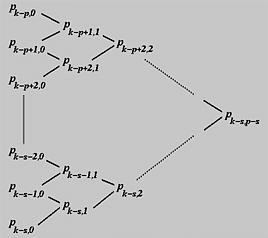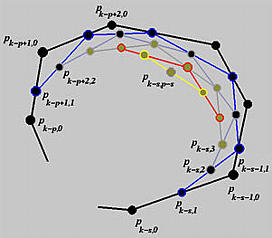While this process looks like the one obtained from de Casteljau's algorithm, in fact they are very different. First, under de Casteljau's algorithm, the dividing points are computed with a pair of numbers 1 - u and u that never change throughout the computation procedure, while under de Boor's algorithm these pairs of numbers are different and depend on the column number and control point number. Second, de Casteljau's algorithm can be used for curve subdivision, while the intermediate control points generated by de Boor's algorithm are not sufficient for this purpose. Third, in de Boor's algorithm only p+1 affected control points are involved in the computation, while de Casteljau's algorithm uses all control points. Since control points pk-p to pk define a convex hull that contains the curve segment on knot span [uk, uk+1), the computation of de Boor's algorithm is performed within the corresponding convex hull.### An Example

Suppose we have a degree 3 B-spline curve (i.e., p = 3) defined by seven control points p0, ..., p6 (i.e., n = 6) and the following knot vector of 11 knots (i.e., m = 10):

 u0 = u1 = u2 = u3 u4 u5 u6 u7 = u8 = u9 = u10 0 0.25 0.5 0.75 1

Based on these information, we shall compute p(0.4). For de Boor's algorithm, this is equivalent to inserting u = 0.4 three times. Since u = 0.4 is not an existing knot, s = 0 and u = 0.4 is in [u4, u5), the affected control points are p4, p3, p2 and p1. The following is the computation scheme: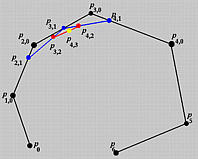Let us compute the second column. The involved a coefficients are

a4,1 = (u - u4) / ( u4+3 - u4) = 0.2
a3,1 = (u - u3) / ( u3+3 - u3) = 8/15 = 0.53
a2,1 = (u - u2) / ( u2+3 - u2) = 0.8
Therefore, the first column is computed as follows:
p4,1 = (1 - a4,1)p3,0 + a4,1p4,0 = 0.8p3,0 + 0.2p4,0
p3,1 = (1 - a3,1)p2,0 + a3,1p3,0 = 0.47p2,0 + 0.53p3,0
p2,1 = (1 - a2,1)p1,0 + a2,1p2,0 = 0.2p1,0 + 0.8p2,0

To compute the second column, we need the following coefficients:

a4,2 = (u - u4) / (u4+3-1 - u4) = 0.3
a3,2 = (u - u3) / (u3+3-1 - u3) = 0.8
The points are
p4,2 = (1 - a4,2) p3,1 + a4,2p4,1 = 0.7p3,1 + 0.3p4,1
p3,2 = (1 - a3,2) p2,1 + a3,2p3,1 = 0.2p2,1 + 0.8p3,1
Finally, since a4,3 = (u - u4)/ (u4+3-2 - u4) = 0.6, the final point is
p4,3 = (1 - a4,3)p3,2 + a4,3p4,2 = 0.4p3,2 + 0.6p4,2
This is the point on the curve corresponding to u = 0.4.

Please notice the similarity of those intermediate polylines generated by de Boor's algorithm and those generated by de Casteljau's algorithm.

Please also note that u is not a knot in this example. If u is a knot, the computation would be easier since we will have fewer number of affected control points.### De Boor's Algorithm for NURBS Curves

De Boor's algorithm also works for NURBS curves. We just multiply every control point by its weight converting the NURBS curve to a 4D B-spline curve, perform de Boor's algorithm on this 4D B-spline curve, and then project the resulting curve back by dividing the first three components with the fourth and keeping the fourth component as its new weight.